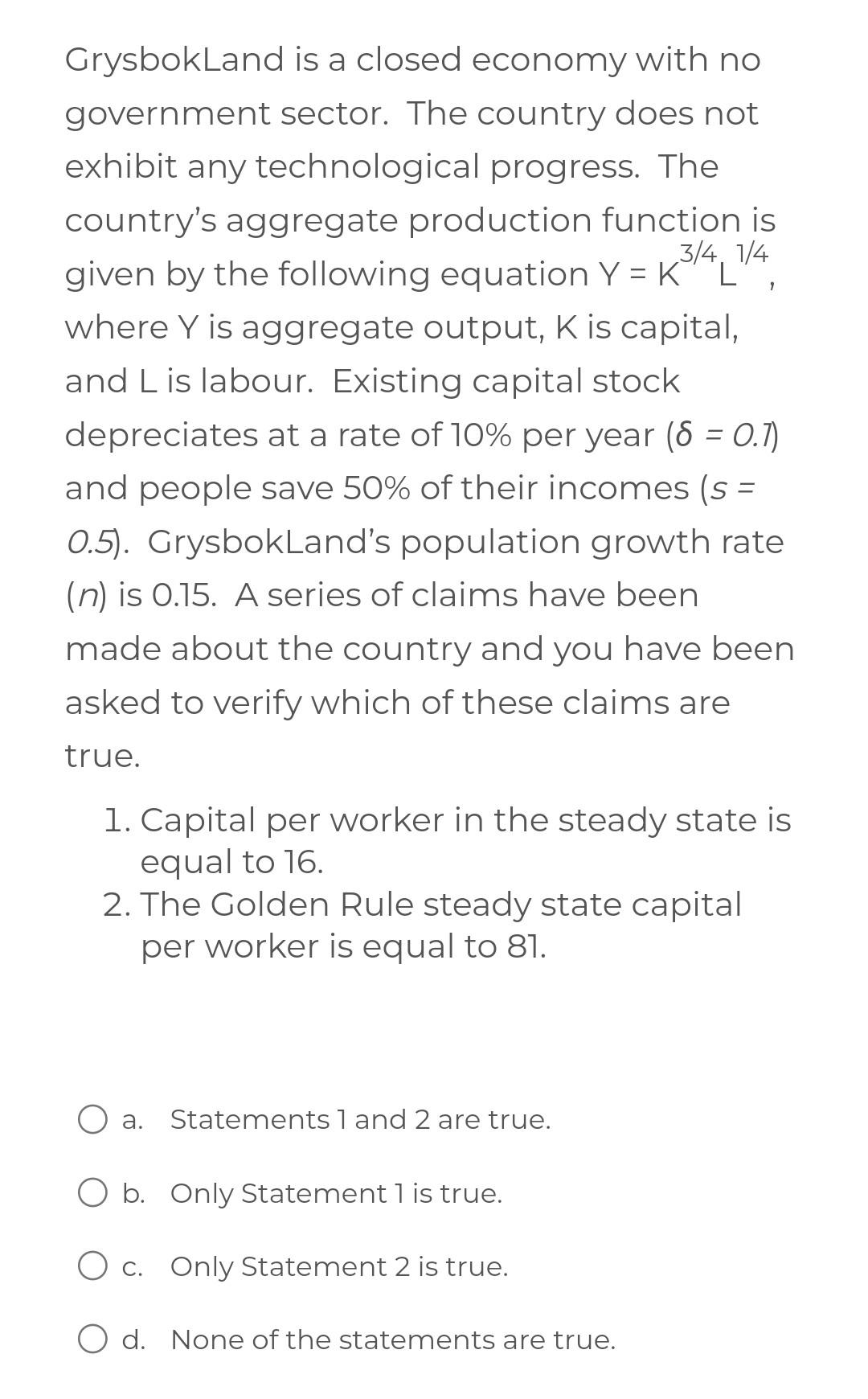Home / Expert Answers / Economics / grysbokland-is-a-closed-economy-with-no-government-sector-the-country-does-not-exhibit-any-tec-pa580

# (Solved): GrysbokLand is a closed economy with no government sector. The country does not exhibit any tec ...GrysbokLand is a closed economy with no government sector. The country does not exhibit any technological progress. The country's aggregate production function is given by the following equation , Where is aggregate output, is capital, and is labour. Existing capital stock depreciates at a rate of per year and people save of their incomes ( 0.5). GrysbokLand's population growth rate is 0.15. A series of claims have been made about the country and you have been asked to verify which of these claims are true. 1. Capital per worker in the steady state is equal to 16. 2. The Golden Rule steady state capital per worker is equal to 81. a. Statements 1 and 2 are true. b. Only Statement 1 is true. c. Only Statement 2 is true. d. None of the statements are true.

We have an Answer from Expert

Ans) option (a) is correct i.e Statement 1 and 2 are true.

We can use the Solow model to determine the steady state values of capital per worker. In the steady state, capital per worker (k) does not change, so we have:
sY = (n + ?)k
where s is the savings rate, Y is output per worker, n is the population growth rate, ? is the depreciation rate, and k is capital per worker.

Substituting Y =     /L and k =    into the above equation, we get:
sK^(3/4)L^(1/4) = (n + ?)K/L
We have an Answer from Expert